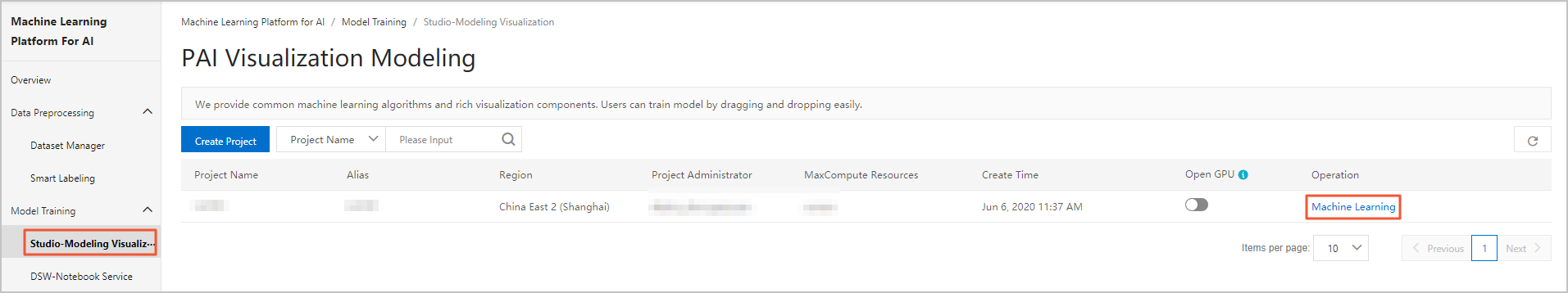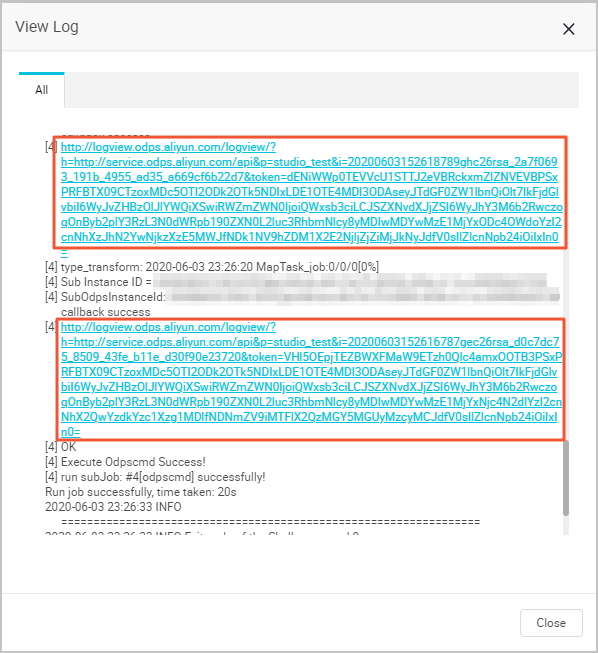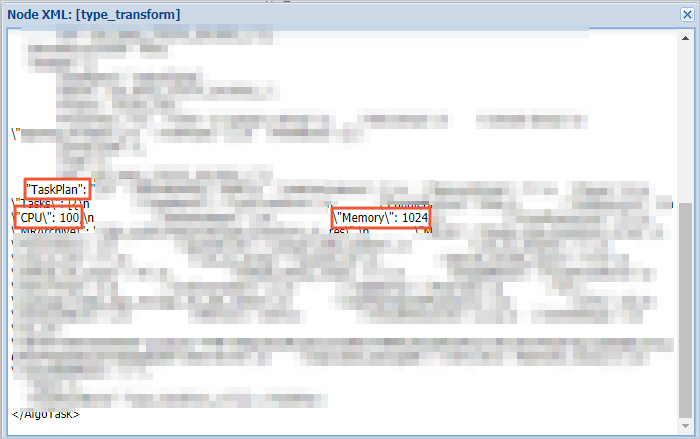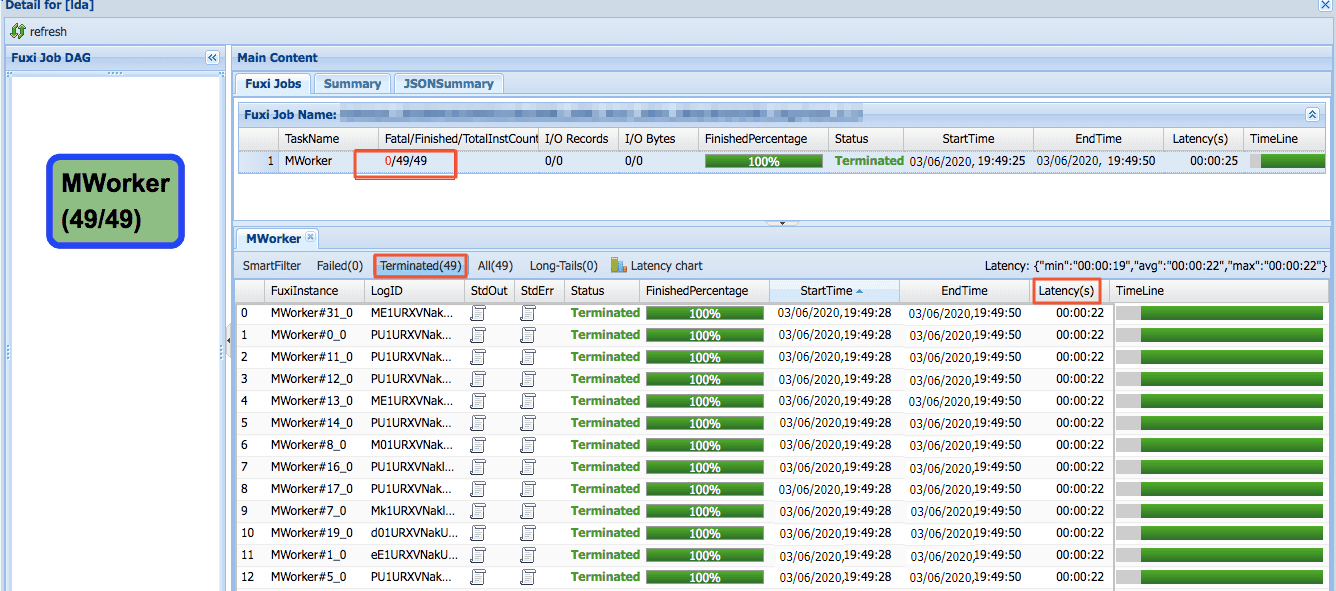In this topic, the Probabilistic Linear Discriminant Analysis (PLDA) component is used as an example to describe how to calculate the fees for experiments running in Machine Learning Studio.

## Background information

An experiment in Machine Learning Studio consists of more than one algorithm component, while an algorithm component is composed of multiple subtasks. To calculate the fees for an experiment, you need to first calculate the costs of subtasks in each algorithm component, then sum up the fees of all components used in the experiment.

## Procedure

1. Determine the category of an algorithm component.
1. Log on to the Machine Learning Platform for AI console.
2. In the left-side navigation pane, choose Model Training > Studio-Modeling Visualization.
3. On the PAI Visualization Modeling page, click Machine Learning in the Operation column.4. On the Algo Platform page, click Components in the left-side navigation pane.
5. In the Components list, find the PLDA component. The PLDA component belongs to the category of text analysis. The component is billed at a price of USD 0.27/compute hour.2. View the resources consumed for running all jobs of a subtask.
1. On the Algo Platform page, click Experiments in the left-side navigation pane.
2. In the My experiments list, click an experiment, and view the experiment flowchart on the canvas.
3. On the canvas, right-click the PLDA component.
4. In the menu that appears, click View Log.7. In the TaskPlan section, view CPU and Memory.• The number of used CPU cores is calculated by dividing the value of CPU by 100. In this example, one CPU core is used for running the job.
• The unit of Memory is MB. In this example, 1024 MB (1 GB) of memory is used for running the job.
8. On the Log Details page, double-click the task below ODPS Task, as shown in the Log details figure.
9. On the Fuxi Jobs tab, click the subtask. In the section that appears, click the Terminated tab. Latency indicates the operation duration of each job.In this example, the subtask has 49 jobs, and each job runs for about 22 seconds.
3. Calculate the fee of the subtask.
1. Calculate the number of compute hours used in the subtask. For more information about how to calculate the number of compute hours, see Billing of Machine Learning Studio.
``The number of compute hours used in the subtask = Max (Number of CPU cores × Usage duration, Memory size × Usage duration/4) = Max [49 × 1 × (22/3,600), 49 × 1 × 22/3,600/4] ≈ 0.30 compute hour``
``Subtask fee = Number of compute hours × Unit price ≈ 0.30 × 0.27 = USD 0.081``